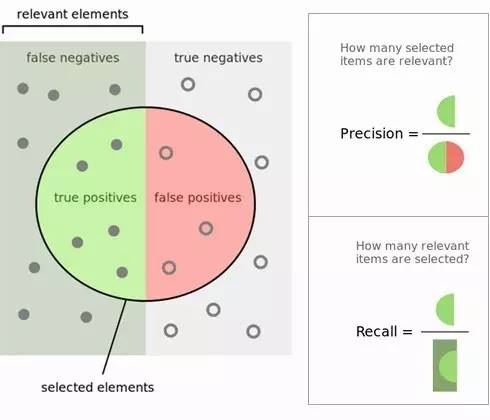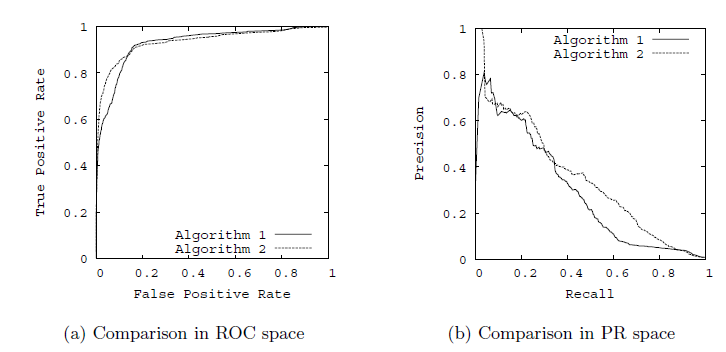# 1. TP, FP, TN, FN

1. 　　True Positives,TP：预测为正样本，实际也为正样本的特征数
2. 　　False Positives,FP：预测为正样本，实际为负样本的特征数
3. 　　True Negatives,TN：预测为负样本，实际也为负样本的特征数
4. 　　False Negatives,FN：预测为负样本，实际为正样本的特征数# 2. 精确率(precision),召回率(Recall)与特异性(specificity)

P=TP/(TP+FP)

R=TP/(TP+FN)

S=FP/(FP+TN)

2/F1=1/P+1/R

$F_\beta=\frac {1+\beta^2*P*R}{\beta^2*P+R}$

# 3. RoC曲线和PR曲线